# RD Sharma Solutions for Class 11 Chapter 15 - Linear Inequations Exercise 15.2

In the previous section, we have learnt how to solve a linear inequation in one variable. In this section, we shall use it to solve a system of linear inequations in one variable. Students who want to get a clear picture of concepts and problem-solving techniques can access the RD Sharma Class 11 Maths Solutions, which is developed by our expert faculty team. The solutions will also help students boost their confidence level and help to score high marks in their board examination. Students can download the pdf easily from the below-mentioned links.

## Download the pdf of RD Sharma Solutions for Class 11 Maths Exercise 15.2 Chapter 15 – Linear Inequations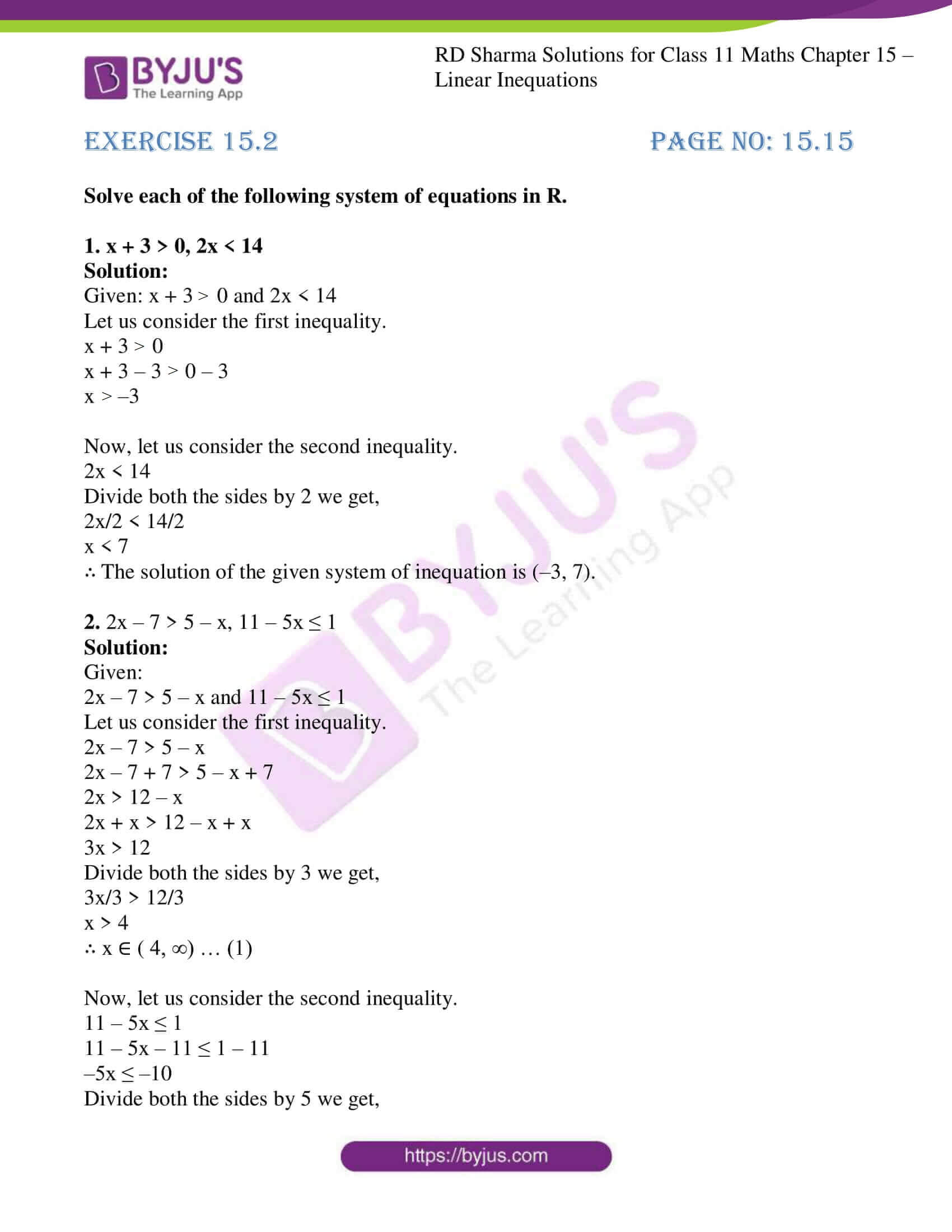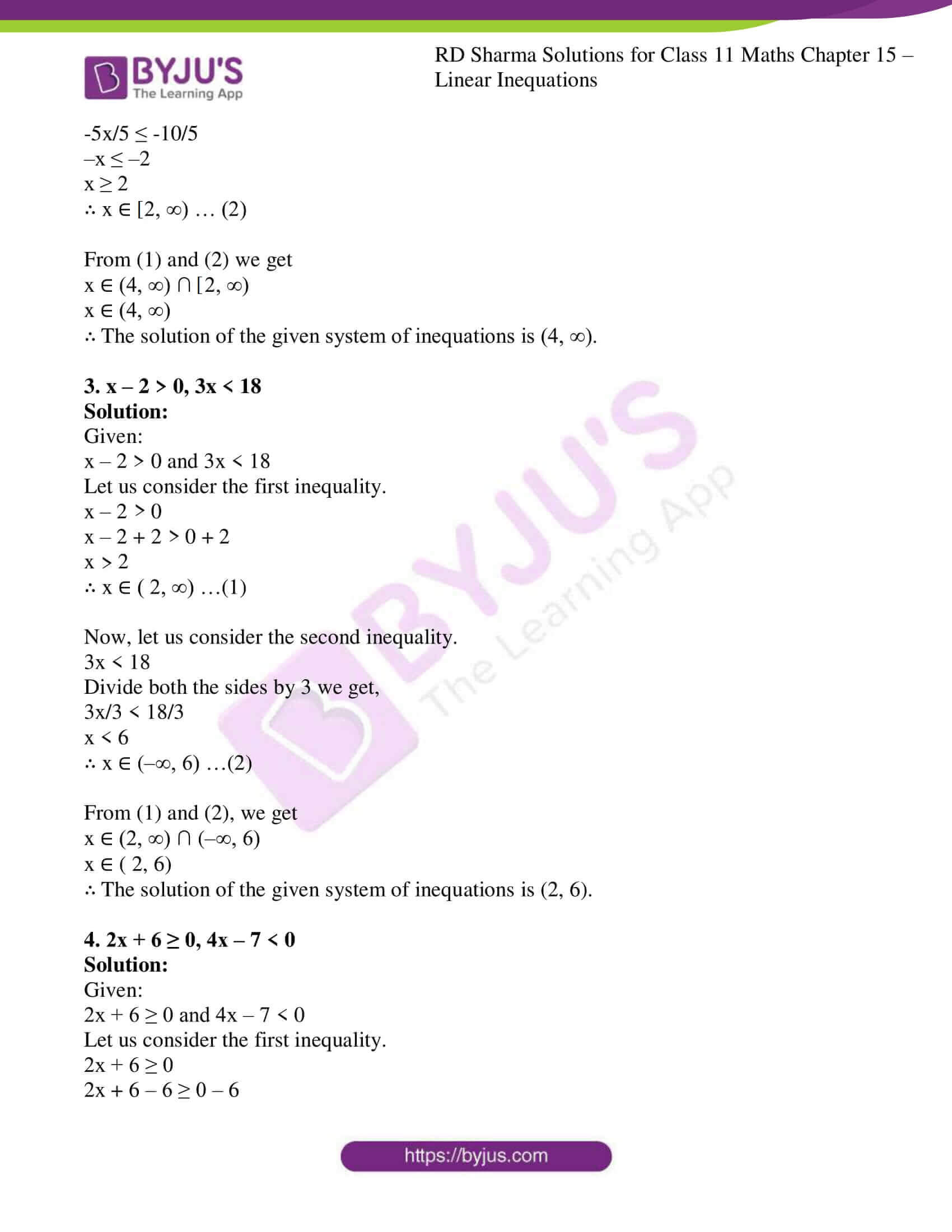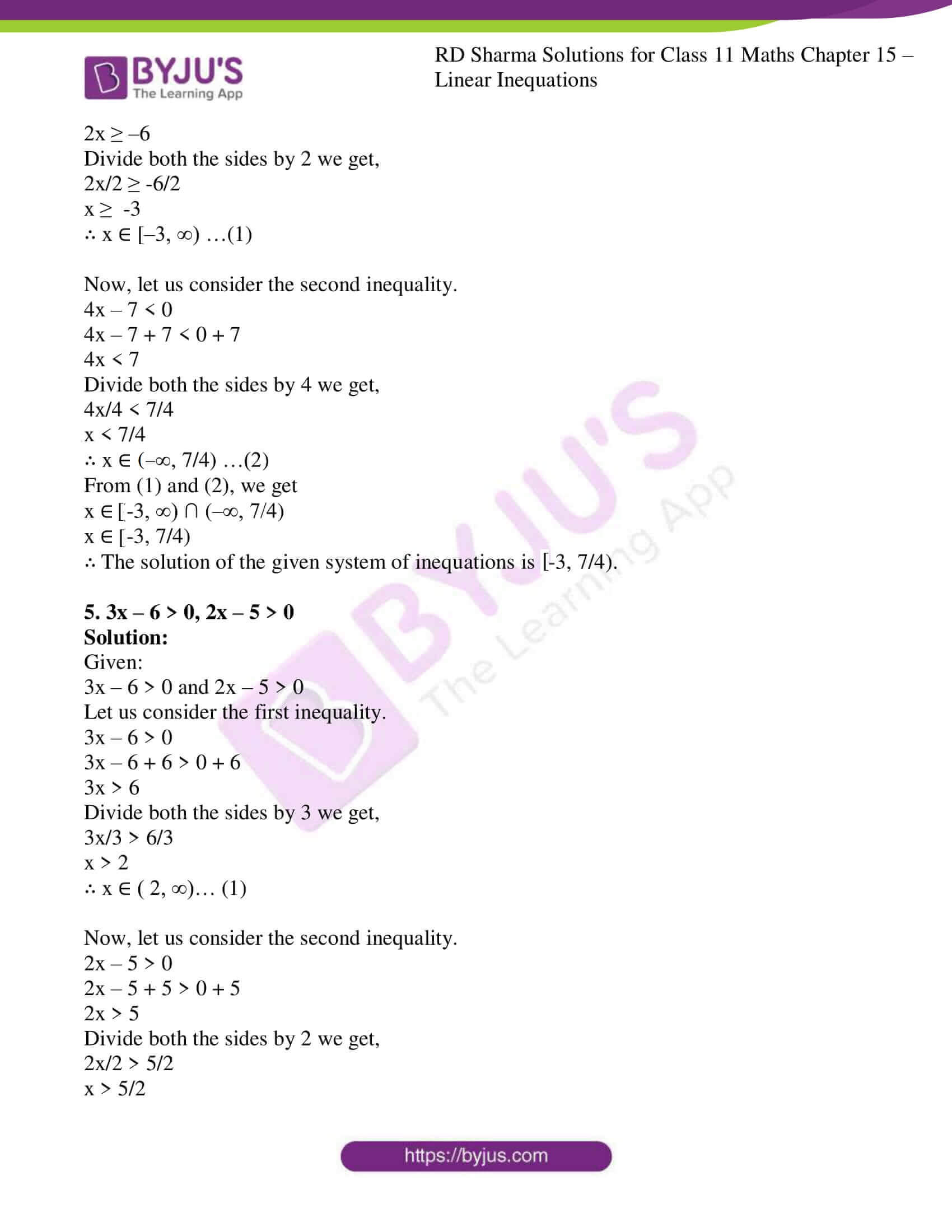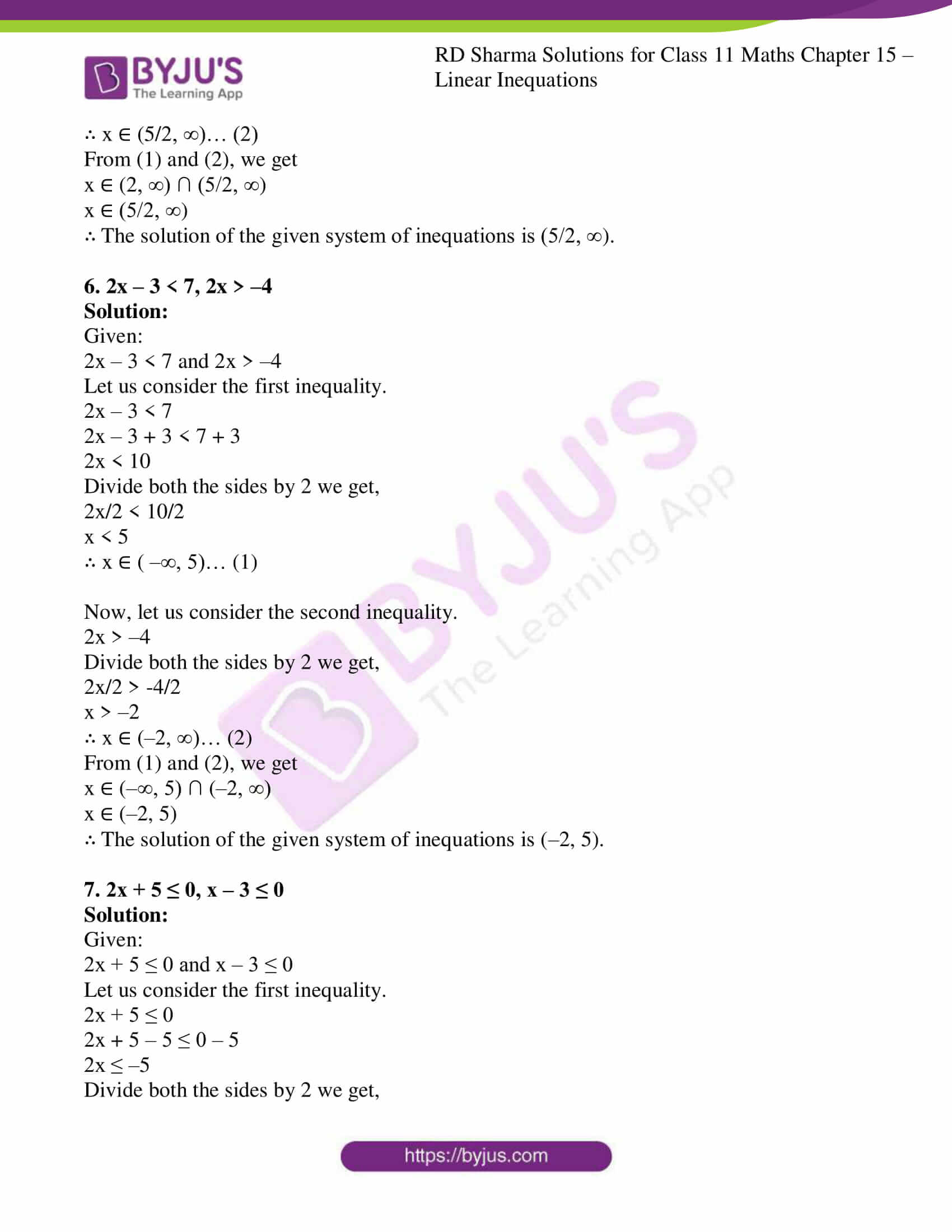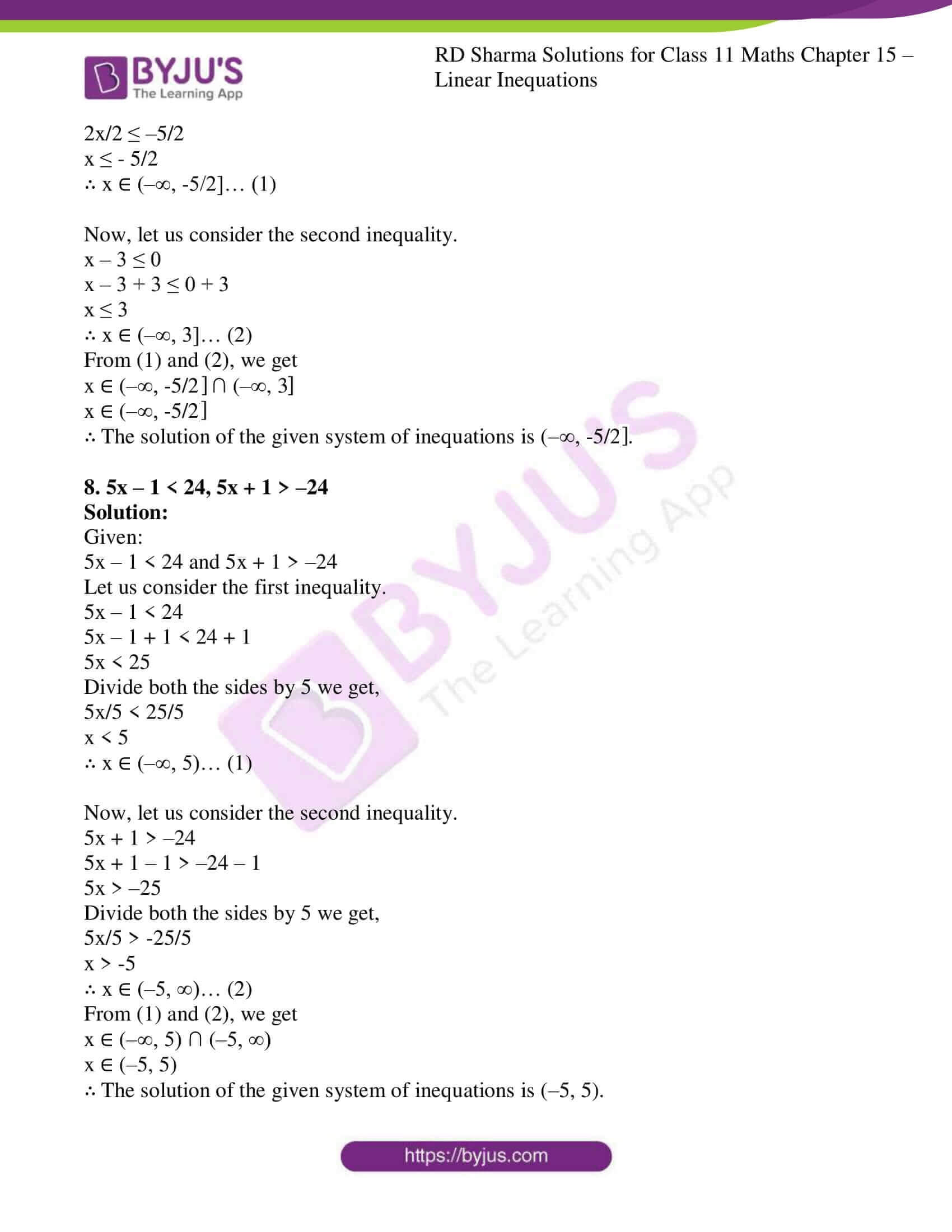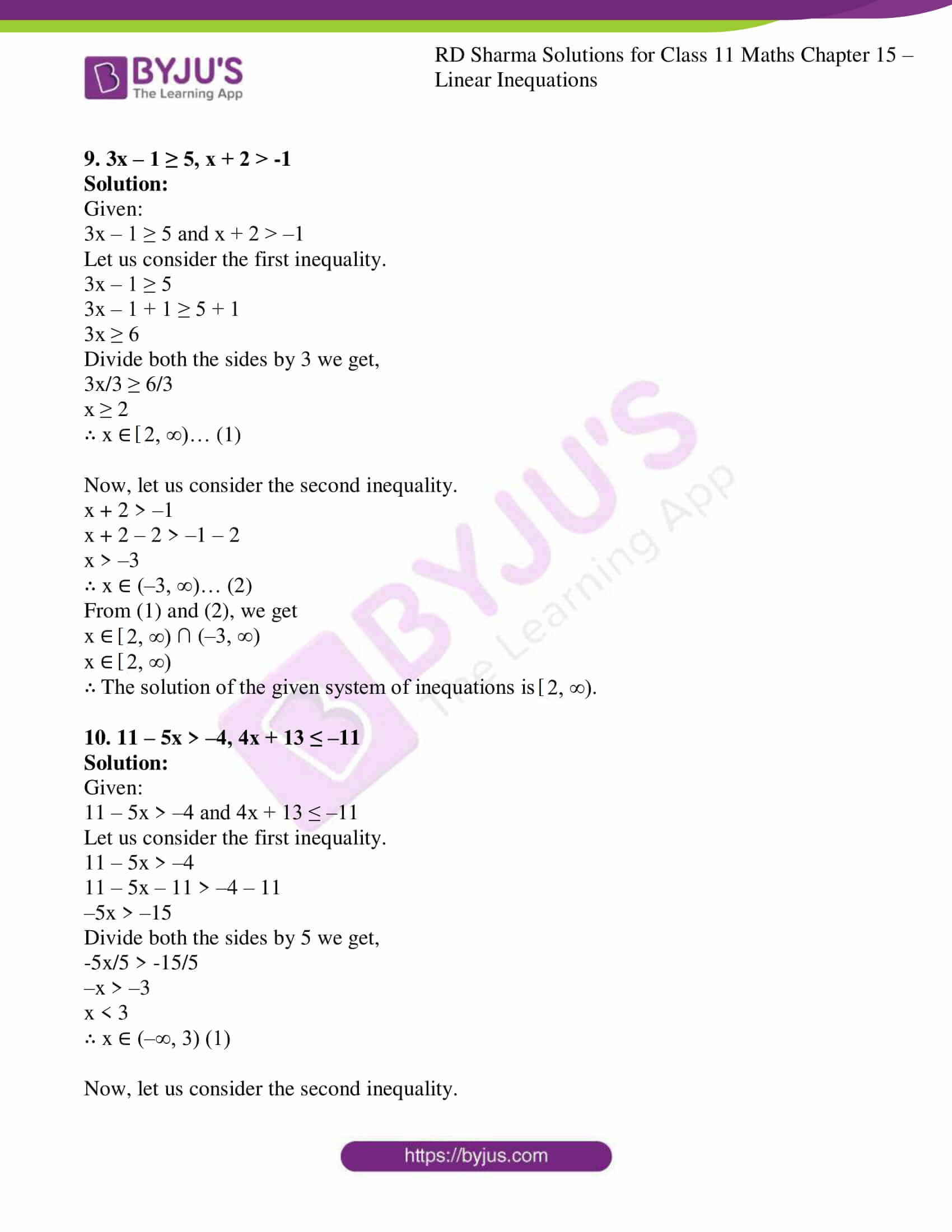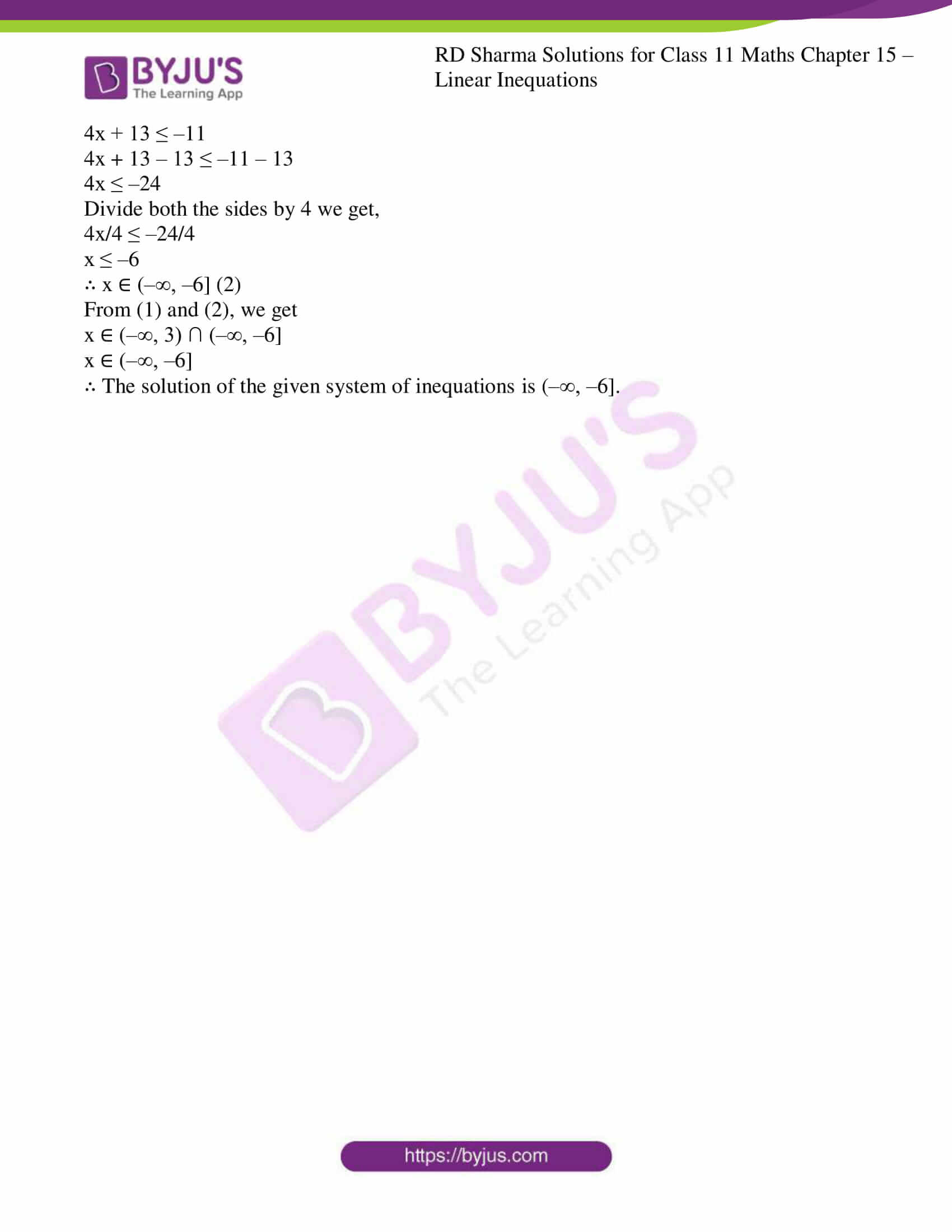### Also, access other exercises of RD Sharma Solutions for Class 11 Maths Chapter 15 – Linear Inequations

Exercise 15.1 Solutions

Exercise 15.3 Solutions

Exercise 15.4 Solutions

Exercise 15.5 Solutions

Exercise 15.6 Solutions

### Access answers to RD Sharma Solutions for Class 11 Maths Exercise 15.2 Chapter 15 – Linear Inequations

Solve each of the following system of equations in R.

1. x + 3 > 0, 2x < 14

Solution:

Given: x + 3 > 0 and 2x < 14

Let us consider the first inequality.

x + 3 > 0

x + 3 – 3 > 0 – 3

x > –3

Now, let us consider the second inequality.

2x < 14

Divide both the sides by 2 we get,

2x/2 < 14/2

x < 7

∴ The solution of the given system of inequation is (–3, 7).

2. 2x – 7 > 5 – x, 11 – 5x ≤ 1

Solution:

Given:

2x – 7 > 5 – x and 11 – 5x ≤ 1

Let us consider the first inequality.

2x – 7 > 5 – x

2x – 7 + 7 > 5 – x + 7

2x > 12 – x

2x + x > 12 – x + x

3x > 12

Divide both the sides by 3 we get,

3x/3 > 12/3

x > 4

∴ x ∈ ( 4, ∞) … (1)

Now, let us consider the second inequality.

11 – 5x ≤ 1

11 – 5x – 11 ≤ 1 – 11

–5x ≤ –10

Divide both the sides by 5 we get,

-5x/5 ≤ -10/5

–x ≤ –2

x ≥ 2

∴ x ∈ [2, ∞) … (2)

From (1) and (2) we get

x ∈ (4, ∞) ∩ [2, ∞)

x ∈ (4, ∞)

∴ The solution of the given system of inequations is (4, ∞).

3. x – 2 > 0, 3x < 18

Solution:

Given:

x – 2 > 0 and 3x < 18

Let us consider the first inequality.

x – 2 > 0

x – 2 + 2 > 0 + 2

x > 2

∴ x ∈ ( 2, ∞) …(1)

Now, let us consider the second inequality.

3x < 18

Divide both the sides by 3 we get,

3x/3 < 18/3

x < 6

∴ x ∈ (–∞, 6) …(2)

From (1) and (2), we get

x ∈ (2, ∞) ∩ (–∞, 6)

x ∈ ( 2, 6)

∴ The solution of the given system of inequations is (2, 6).

4. 2x + 6 ≥ 0, 4x – 7 < 0

Solution:

Given:

2x + 6 ≥ 0 and 4x – 7 < 0

Let us consider the first inequality.

2x + 6 ≥ 0

2x + 6 – 6 ≥ 0 – 6

2x ≥ –6

Divide both the sides by 2 we get,

2x/2 ≥ -6/2

x ≥ -3

∴ x ∈ [–3, ∞) …(1)

Now, let us consider the second inequality.

4x – 7 < 0

4x – 7 + 7 < 0 + 7

4x < 7

Divide both the sides by 4 we get,

4x/4 < 7/4

x < 7/4

∴ x ∈ (–∞, 7/4) …(2)

From (1) and (2), we get

x ∈ [-3, ∞) ∩ (–∞, 7/4)

x ∈ [-3, 7/4)

∴ The solution of the given system of inequations is [-3, 7/4).

5. 3x – 6 > 0, 2x – 5 > 0

Solution:

Given:

3x – 6 > 0 and 2x – 5 > 0

Let us consider the first inequality.

3x – 6 > 0

3x – 6 + 6 > 0 + 6

3x > 6

Divide both the sides by 3 we get,

3x/3 > 6/3

x > 2

∴ x ∈ ( 2, ∞)… (1)

Now, let us consider the second inequality.

2x – 5 > 0

2x – 5 + 5 > 0 + 5

2x > 5

Divide both the sides by 2 we get,

2x/2 > 5/2

x > 5/2

∴ x ∈ (5/2, ∞)… (2)

From (1) and (2), we get

x ∈ (2, ∞) ∩ (5/2, ∞)

x ∈ (5/2, ∞)

∴ The solution of the given system of inequations is (5/2, ∞).

6. 2x – 3 < 7, 2x > –4

Solution:

Given:

2x – 3 < 7 and 2x > –4

Let us consider the first inequality.

2x – 3 < 7

2x – 3 + 3 < 7 + 3

2x < 10

Divide both the sides by 2 we get,

2x/2 < 10/2

x < 5

∴ x ∈ ( –∞, 5)… (1)

Now, let us consider the second inequality.

2x > –4

Divide both the sides by 2 we get,

2x/2 > -4/2

x > –2

∴ x ∈ (–2, ∞)… (2)

From (1) and (2), we get

x ∈ (–∞, 5) ∩ (–2, ∞)

x ∈ (–2, 5)

∴ The solution of the given system of inequations is (–2, 5).

7. 2x + 5 ≤ 0, x – 3 ≤ 0

Solution:

Given:

2x + 5 ≤ 0 and x – 3 ≤ 0

Let us consider the first inequality.

2x + 5 ≤ 0

2x + 5 – 5 ≤ 0 – 5

2x ≤ –5

Divide both the sides by 2 we get,

2x/2 ≤ –5/2

x ≤ – 5/2

∴ x ∈ (–∞, -5/2]… (1)

Now, let us consider the second inequality.

x – 3 ≤ 0

x – 3 + 3 ≤ 0 + 3

x ≤ 3

∴ x ∈ (–∞, 3]… (2)

From (1) and (2), we get

x ∈ (–∞, -5/2] ∩ (–∞, 3]

x ∈ (–∞, -5/2]

∴ The solution of the given system of inequations is (–∞, -5/2].

8. 5x – 1 < 24, 5x + 1 > –24

Solution:

Given:

5x – 1 < 24 and 5x + 1 > –24

Let us consider the first inequality.

5x – 1 < 24

5x – 1 + 1 < 24 + 1

5x < 25

Divide both the sides by 5 we get,

5x/5 < 25/5

x < 5

∴ x ∈ (–∞, 5)… (1)

Now, let us consider the second inequality.

5x + 1 > –24

5x + 1 – 1 > –24 – 1

5x > –25

Divide both the sides by 5 we get,

5x/5 > -25/5

x > -5

∴ x ∈ (–5, ∞)… (2)

From (1) and (2), we get

x ∈ (–∞, 5) ∩ (–5, ∞)

x ∈ (–5, 5)

∴ The solution of the given system of inequations is (–5, 5).

9. 3x – 1 ≥ 5, x + 2 > -1

Solution:

Given:

3x – 1 ≥ 5 and x + 2 > –1

Let us consider the first inequality.

3x – 1 ≥ 5

3x – 1 + 1 ≥ 5 + 1

3x ≥ 6

Divide both the sides by 3 we get,

3x/3 ≥ 6/3

x ≥ 2

∴ x ∈ [2, ∞)… (1)

Now, let us consider the second inequality.

x + 2 > –1

x + 2 – 2 > –1 – 2

x > –3

∴ x ∈ (–3, ∞)… (2)

From (1) and (2), we get

x ∈ [2, ∞) ∩ (–3, ∞)

x ∈ [2, ∞)

∴ The solution of the given system of inequations is [2, ∞).

10. 11 – 5x > –4, 4x + 13 ≤ –11

Solution:

Given:

11 – 5x > –4 and 4x + 13 ≤ –11

Let us consider the first inequality.

11 – 5x > –4

11 – 5x – 11 > –4 – 11

–5x > –15

Divide both the sides by 5 we get,

-5x/5 > -15/5

–x > –3

x < 3

∴ x ∈ (–∞, 3) (1)

Now, let us consider the second inequality.

4x + 13 ≤ –11

4x + 13 – 13 ≤ –11 – 13

4x ≤ –24

Divide both the sides by 4 we get,

4x/4 ≤ –24/4

x ≤ –6

∴ x ∈ (–∞, –6] (2)

From (1) and (2), we get

x ∈ (–∞, 3) ∩ (–∞, –6]

x ∈ (–∞, –6]

∴ The solution of the given system of inequations is (–∞, –6].